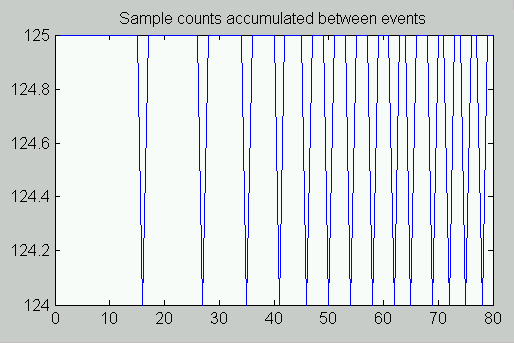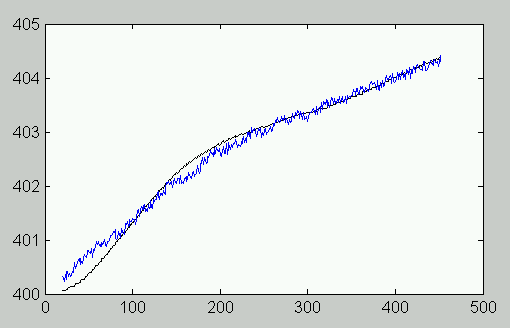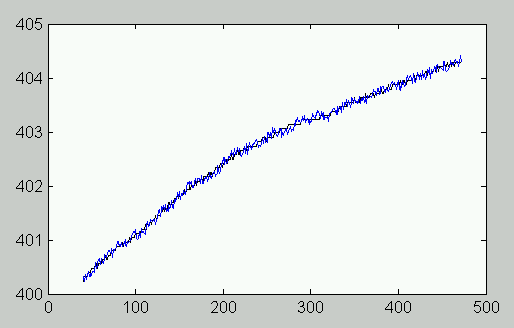# Engine Speed Monitoring: The Alpha-Beta Filter

## Introduction(View more Control information or download the preliminary implementation.)

Estimating an engine's speed is not trivial, particularly when the only information available is a "top dead center" pulse that occurs once per rotation. While the encoder can produce this pulse at any time, its detection is delayed until the next sampling instant. The speed is continually disrupted by disturbances from a chaotic combustion process. A simple speed estimate, obtained from the time interval between samples of "top dead center events," might be too erratic for speed control applications.

When considering how to best estimate the current dynamic state of a system in the presence of noise, the first thing that might come to mind is a Kalman filter . There are some clear motivations for this. The engine dynamics are well described by linear equations. Combustion disturbances influence the dynamic states, and there are numerous problems getting clean measurements in the presence of high-power discharges. The Kalman filter models both kinds of disturbances explicitly. But setting up a Kalman filter requires a large investment in engineering time for developing an accurate system model. Somehow this seems like overkill for determining how fast something turns.

So consider where some simplifications might occur.

#### Simplifications in the Dynamic Model

Dynamics of an engine system involve rotation angle, speed, and acceleration. This is a very low order system with an especially simple cascaded structure. Velocity might ramp up or down slightly over a few revolutions, but not much. A completely general state transition matrix model is more complicated than what is really necessary to represent the system dynamics.

#### Simplifications in the Stochastic Model

A Kalman filter model requires models for state and measurement noise. We can ignore the distinction and avoid developing these explicit noise models.

#### Relaxation of Optimality

A Kalman filter guarantees (under assumptions of a exactly known perfectly linear system with exactly known noise statistics) a minimum-variance state estimate. At the end of the day, however, a noisy measurement remains noisy. A near-optimal result is often good enough.

If the noise processes change, and this change is reflected in the Kalman filter noise model, it is able to adapt to the new conditions and maintain optimality. If you don't need the adaptive capability, you can avoid its complexities by fixing the gains at known good values, without waiting for the Kalman updates to compute them iteratively.

## The Alpha-Beta Filter

Assume for the moment that the system dynamics consist of two discrete-time equations. These dynamic equations should yield perfect predictions of future angular positions, assuming constant angular velocity undisturbed by noise.

```  velocity is constant
v+  <--  v

angular position increase
proportional to constant velocity
x+  <--  x + (ΔT) v
```

Here, the "plus subscript" notation means the predicted future value.

We can observe measured values of `x`, calling these `xmeas` and compare to the predictions. Because the measurements are noisy, the actual angular position `x` is not equal to the measurement, but on the other hand, a measurement might indicate something about error in the predicted state. The Kalman gain would tell us precisely how much the prediction should be adjusted to improve the estimates and reduce variance. Calling the Kalman gain terms for angular position and velocity alpha and beta respectively, the correction terms are added to the original dynamic equations to produce the complete filter.

```  angular position prediction
xp  <--  x + (ΔT) v

adjusted angular position estimate
x+  <--  xp + α (xmeas - xp)

v+  <--  v + β (xmeas - xp)
```

For the next cycle, the "plus" terms assume the roles of the unsubscripted terms on the right, and the process repeats.

But of course, we do not have the Kalman filter equations to compute for us the correction gain values alpha and beta. So we supply good values. This is the Alpha-Beta filter .

## Alpha-Beta Filtering and State Observers

Regular visitors at this web site will probably note that there are a lot of similarities between the Alpha-Beta filtering and the state observer , as described in another note. The difference is... there is no difference. Though the Alpha-Beta filtering strategy derives from Kalman filtering, what results is really just a special form of the state observer filter.

## A Generalization for Ramping Speed

If engines are spinning really fast, there are lots of "top dead center" events, occurring frequently. They occur so fast that there is very little time for anything to change, so the assumption of constant velocity is almost perfect. Any tiny discrepancy will be indistinguishable from the noise disturbance and corrected along with it.

For slower speeds, however, it is possible to see the consistent effects of "ramping" speed change as the engine accelerates. To allow for this, we can extend the dynamic equations slightly. Instead of assuming constant speed, assume constant acceleration. We know that under ideal conditions with constant acceleration, the dynamic equations would be

```  angular position change
x+  <--  x + (ΔT) v + 1/2 (ΔT)2 a

angular velocity change
v+  <--  v + (ΔT) a

acceleration is constant
a+  <--  a
```

Clearly, if the unknown acceleration estimate is too low, both the velocity and the angular position estimates will also be too low. We need a "Kalman gain" correction for this new term as well. We will assign new gain value `gamma` for this term. The extended Alpha-Beta filter, sometimes called an Alpha-Beta-Gamma filter, can be defined as

```  prediction  <--  x + (ΔT) v + 1/2 (ΔT)2 a
change  <-- (xmeas - prediction)
x+  <--  prediction + α change
v+  <--  v + (ΔT) a + β change
a+  <--  a + γ change
```

This follows the classic formulation of Alpha-Beta filtering, but when applied to engine speed, this formulation has problems. We will discuss why and provide a solution in the next section.

## Measuring Engine Speed: Inverse Prediction

Unfortunately, the direct formulation fails to match the requirements of the engine speed application. The first equation has a fixed `ΔT` interval for estimating a changing rotation angle. But for the engine application, what we have instead is a unknown changing `ΔT` and a fixed, enforced rotation angle – often one full rotation cycle per interval. We have to attack the problem from the reverse direction.

We know the rotation angle of the next event exactly. Say that it is one complete rotation. Substituting this known fixed change in rotation angle, and starting each rotation independently, the state equations can be rearranged.

```  a+ (ΔT)  <--  a (ΔT)

v+  <--  v  +  a (ΔT)

(x+ - x) / (ΔT)  <--   v + 1/2 a (ΔT)
```

We can note that the `(x+ - x)` term is the fixed and unchanging rotation angle. If we now define two new variables P and Q as follows:

```      P  =  (x+ - x) / (ΔT)
Q  =   a (ΔT)
```

then the modified filter equations take the following form using these two new variables.

```  Q+  <--  Q

v+  <--  v  +  Q

P+  <--  v  +  1/2 Q
```

This has the same sort of cascaded structure as before, so the same kind of Alpha-Beta correction strategy can be applied. One thing different about these equations, the P term does not appear on the right hand side as angular position x did. We can stabilize changes to this variable by lagging its computed value. This yields the modified Alpha-Beta filtering equations:

```  change = (fixed rotation angle)/ΔT - ( v + 1/2 Q )
P+  <--  0.8*P + 0.2*( v + 1/2 Q ) + α * change;
v+  <--  v  +  Q + β * change;
Q+  <--  Q + γ * change;
```

## Choosing Gains

Values of the gain terms must be selected and verified experimentally. However, this is not as hard as it sounds, because you can start from reasonable initial values and adjust from there.

The value of beta will be moderately small so that speed estimates are not unduly affected by variations from input data. A typical value is around 0.05 to 0.10.

The gamma gain term helps to track changes with less sensitivity to input data, and a typical value is usually around 0.005. The alpha correction is not related directly to the kinematic equations, but it helps to enforce consistency between the velocity and acceleration variables. A typical value is around 0.10 to 0.20.

The default alpha-beta-gamma gains built into the filter implementation are unlikely to work best for all applications, so start with gains in the middle of the suggested ranges and adjust appropriately.

## An Implementation: the ENGSPEED command

An implementation of the Alpha-Beta filter for engine speed monitoring has been provided in the `ENGSPDM.DLM` downloadable module, available on the download page. This module has the following special features:

• Default tuning. Default gain values are built in, but alternate tunings can be provided as desired.
• Slewing mode. When the number of samples changes drastically from one rotation event to the next, the computations would not be accurate anyway. The speed estimates are forced to `1/ΔT` and the usual filter updates are bypassed.
• Unit adjustments. The sampling time `dT` in seconds and the number of equally-spaced rotation events per cycle `N` are specified. This allows the command to scale the results to RPM units.
• Multi-channel support. Usually, in addition to speed monitoring, several data channels will be sampled and recorded. Signals are collected and reported together to the host system.
• Interpolated output. The command uses the filter state to project the current velocity during the intervals between encoder pulses. The current speed estimate is multiplexed with the channel measurements, making the data easy to log.

For example, suppose that an application is configured to sample five channels, four data channels, and one "top dead center" rotation event pulse. To ensure that the event pulse is detected as quickly as possible, the encoder signal is sampled alternately with each of the data channels. There is one "top and center" event pulse per rotation. The timing is set so that the data channels are sampled in 40 microsecond cycles, with 5 microseconds to check for the event pulse plus 5 microseconds to sample a data channel, repeated 4 times.

The input sampling configuration for this application is:

```  IDEFINE  ENGINE4
CHANNELS 8
SET IP0  S0     // Event detection
SET IP1  S1     // Signal 1
SET IP2  S0     // Event detection
SET IP3  S2     // Signal 2
SET IP4  S0     // Event detection
SET IP5  S3     // Signal 3
SET IP6  S0     // Event detection
SET IP7  S4     // Signal 4
TIME 5
END
```

The processing application is as follows:

```  PDEFINE  CAPTURE
ENGSPEED(IP(0..7),4,  0,  40.0e-6,  1,  \$binout)
END
```

The output stream has five multiplexed channels, the first being the current best estimate of the engine speed and the rest being the measurements.

## Results

The actual input data the filter is sees is sparse data that bounces around between fixed integer levels. How many samples of equal time duration occurred during one rotation cycle? This example shows between 124 and 125 samples per rotation event, indicating a speed somewhere in the range 400.0 to 403.2 RPM.The following plot shows the response of the engine speed filter, superimposed on the actual underlying velocities prior to sampling.Some observations:

• The filter has an obvious transient behavior. It will react to unexpected changes in acceleration.
• In quiet periods with little change in sample counts, filter dynamics follow a ramped course.
• Deviations are small after transients settle.
• Updates are applied upon occurrence of each encoder event.
• Between events, internal dynamics are used to interpolate the speed trajectory.

It is instructive to compare this to results of applying a FIR filter to the sample count data, such as the following, using RAVERAGE with a 40 sample window.• There is a big advantage to knowing what the future holds. The quality of the speed estimates is distinctly better.
• The running average filter is higher order so it tracks transient effects much more closely. This can be an advantage or disadvantage.
• Where Alpha-Beta estimates are updated instantly, the running average filter must delay until both "past data" and "future data" are available. For an engine at 400 RPM, as in this example, the delay is an additional 2.8 seconds; at 60 RPM, it is an additional 18.6 seconds. Control applications might not be able to tolerate such delays.
• The running average filter provides no supplementary information for interpolating along the velocity trajectory.

## Conclusion

Smoothing encoder pulsed signals to determine engine speed, producing the needed information with minimum delay, is a non-trivial problem. Kalman filters and their simplified Alpha-Beta variants can serve as speed estimators, smoothing chattering signals to produce current estimates required for control applications.

Despite all of the background technology, Alpha-Beta filtering boils down to a simple set of equations easily packaged as the `ENGSPEED ` processing command that you can use with your Data Acquisition Processor (DAP) board system. Updated speed estimates are available within milliseconds of the arrival of each timing pulse. Interpolated speed estimates are available at all times between. Accurate estimates, fast response, and reliability of DAP operation, in addition to the data capture and logging processes supported simultaneously, make the `ENGSPEED` command a useful tool for engine speed monitoring and control applications.

#### Footnotes and References:

1. An Introduction to the Kalman Filter, Greg Welch and Gary Bishop. Technical report TR95-041, 1995. Published by University of North Carolina at Chapel Hill, Chapel Hill, NC, USA. This is perhaps the clearest available introductory survey of Kalman filtering, with copies posted in numerous locations on the Internet.
2. The Alpha-Beta Filter, Robert Penoyer, C User's Journal, Vol. 11, No. 7, pp. 73-86, 1993. This is an easy introduction, available on the CD-ROM issues. Provides a useful textbook and application paper bibliography as well.
3. DAPL Commands as State Observers - A Hydraulic Control Application, on this site. Hydraulic control is clearly much different than engine speed monitoring, but the discussion of how state observers work in general is relevant.